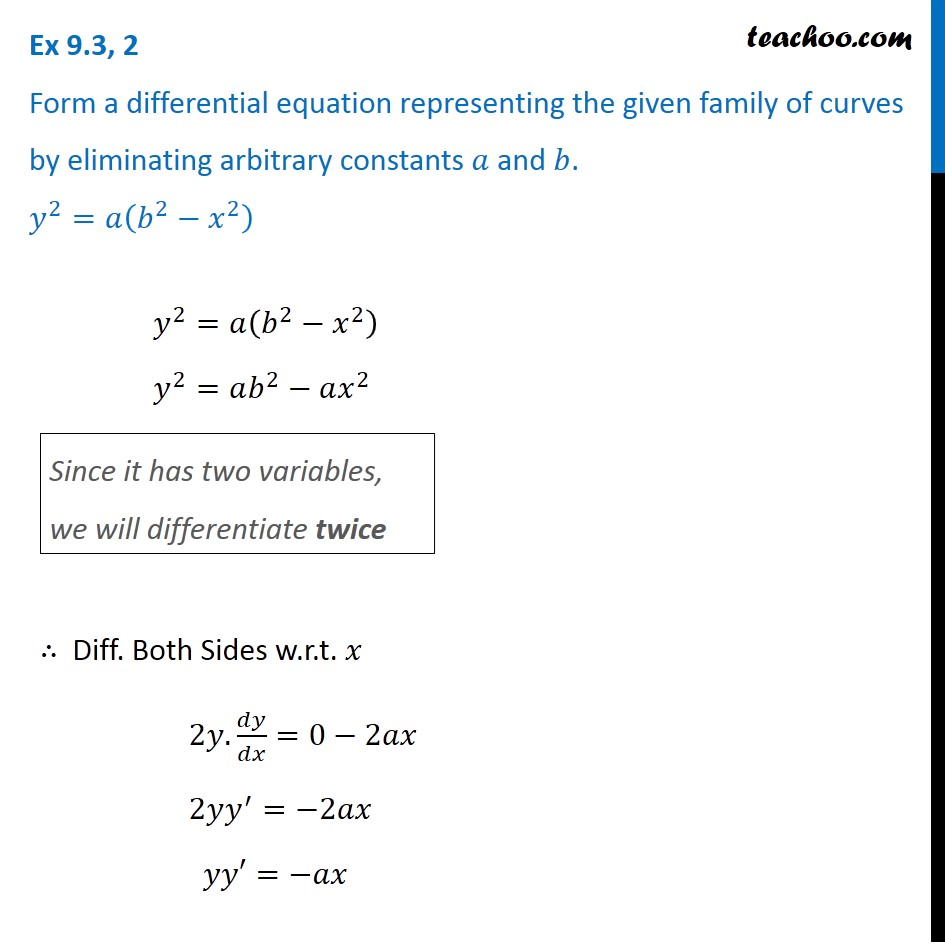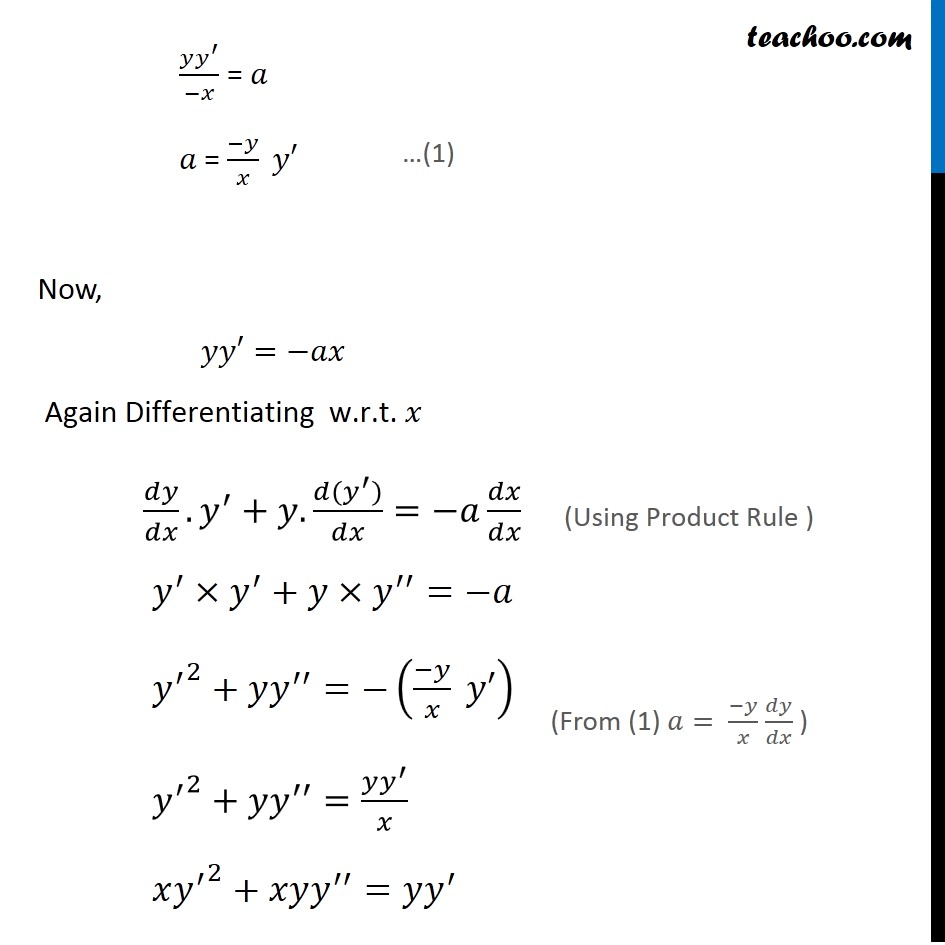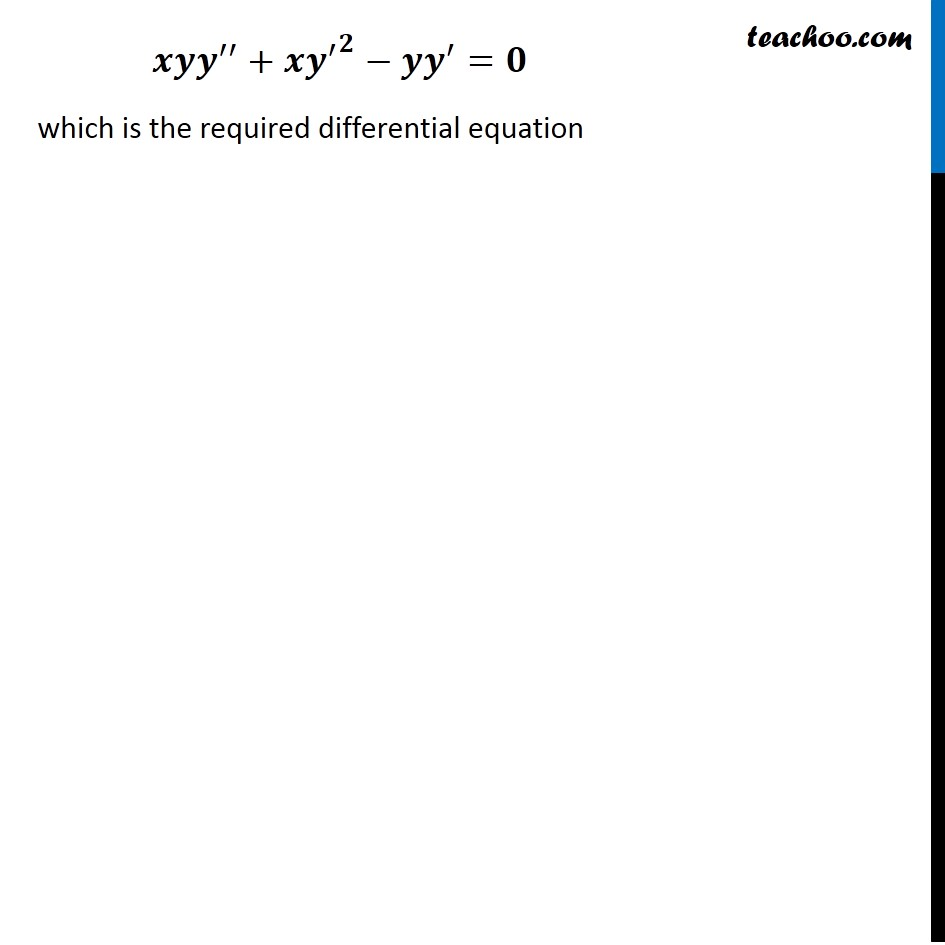Forming Differential equations

Chapter 9 Class 12 Differential Equations
Serial order wiseLearn in your speed, with individual attention - Teachoo Maths 1-on-1 Class

### Transcript

Question 2 Form a differential equation representing the given family of curves by eliminating arbitrary constants 𝑎 and 𝑏. 𝑦^2=𝑎(𝑏^2−𝑥^2 ) 𝑦^2=𝑎(𝑏^2−𝑥^2 ) 𝑦^2=𝑎𝑏^2−𝑎𝑥^2 Since it has two variables, we will differentiate twice ∴ Diff. Both Sides w.r.t. 𝑥 2𝑦.𝑑𝑦/𝑑𝑥=0−2𝑎𝑥 2𝑦𝑦^′=−2𝑎𝑥 𝑦𝑦′=−𝑎𝑥 (𝑦𝑦^′)/(−𝑥) = 𝑎 𝑎 = (−𝑦)/𝑥 𝑦′ Now, 𝑦𝑦′=−𝑎𝑥 "Again Differentiating w.r.t. " 𝑥 𝑑𝑦/𝑑𝑥.𝑦^′+𝑦.(𝑑(𝑦^′))/𝑑𝑥=−𝑎 𝑑𝑥/𝑑𝑥 𝑦^′×𝑦^′+𝑦×𝑦^′′=−𝑎 〖𝑦^′〗^2+𝑦𝑦^′′=−((−𝑦)/𝑥 𝑦′) 〖𝑦^′〗^2+𝑦𝑦^′′=(𝑦𝑦^′)/𝑥 𝑥〖𝑦^′〗^2+𝑥𝑦𝑦^′′=𝑦𝑦^′ …(1) ("Using Product Rule ") (From (1) 𝑎= (−𝑦)/𝑥 𝑑𝑦/𝑑𝑥 ) 𝒙𝒚𝒚^′′+𝒙〖𝒚^′〗^𝟐−𝒚𝒚^′=𝟎 which is the required differential equation